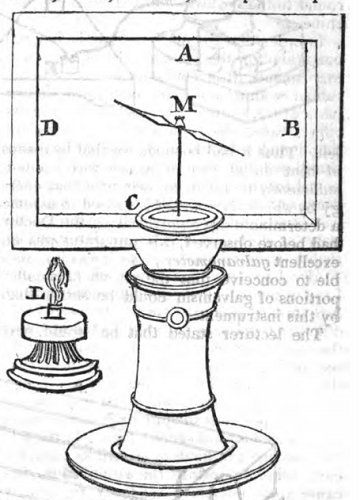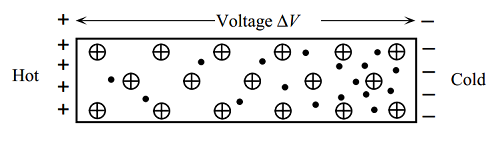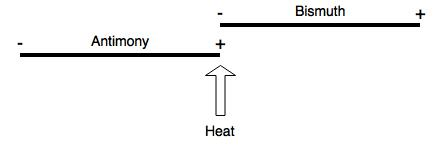# Seebeck Effect

#### Discovery of Seebeck EffectThe idea of electricity generation was first found by Thomas Johann Seebeck in 1823 (See The London Mechanic’s Register, P.406). By putting bismuth and antimony in an alternating manner to form a rectangle (See picture above) and heating one corner, Seebeck was able to deflect a compass placed in the middle of the rectangle.

#### Drude Model and Sommerfeld TheoryWith classical theory, from the Drude model of solid (See the figure above), the charge carriers (electrons for metals and holes for p-type semiconductors) move from hot side to cold side. A more detailed derivation of the seebeck coefficient, with Fermi-dirac Distribution, is given in the first section of this document: http://electronicmaterials.usask.ca/Samples/Thermoelectric-Seebeck.pdf

The formula agreed wit some metals like Na, K, Al. But it does not match with the experimental data of some other metals, sometimes even the sign of seebeck coefficient is different. A more complicated Mott expression can be used to calculate the coefficient (refer to P.19 of Thermoelectric Power of Metals)

#### ThermocoupleWhen two dissimilar metals of opposite signs of Seebeck coefficients, the two metals combined to act like a battery. For example, from the diagram above showing a pair of bismuth and antimony, if the junction of two dissimilar metals are heated, under different seebeck coefficient of the two metals (+47 uV/K and -72uV/K), a seebeck voltage will generate across the two metals because of the difference in coefficients.

#### Thermo”pile” by Joseph Henry

For the case of bismuth and antimony, which is the combination Joseph Henry used in his experiment of sunspot, have seebeck coefficient of +47uV/K and -72uV/K. This will generate the greatest seebeck voltage using metals (From Electronic Coolings, http://www.electronics-cooling.com/2006/11/the-seebeck-coefficient/). By having, for instance, ten couples of bismuth and antimony like the figure below, will generate a great voltage that deflects a microammeter significantly.For the thermopile used by Joseph Henry(see “Henry apparatus”) , the number of couples is 25 plates of bismuth and antimony joined in a “zig-zag” way. So roughly speaking (because Seebeck coefficient is actually temperature dependent), the sensitivity of the thermopile is 25*(47+72)=2.975mV/K.

#### Joseph Henry’s list of thermoelectrical relation:

In Joseph Henry lecture in 1846, according to the class notes taken by Henry C. Cameron, a table of the thermal electrical relations from extreme positive to extreme negative is recorded. And the corresponding Seebeck Coefficients, measured in modern time, is summarized in the table attached here:

Table-of-Seebeck-Coefficients-JH.pdf

In general, the list given by Joseph Henry has discrepancies with modern measurement.

#### Solid State Physics for Seebeck Effect

In order to understand the Seebeck Effect, first principles obtained from solid state physics regarding metals are needed. A review of Drude model to Semi-classical transport is created to understand the device.

Joseph-Henry-Project-Thermoelectric-Battery.pdf